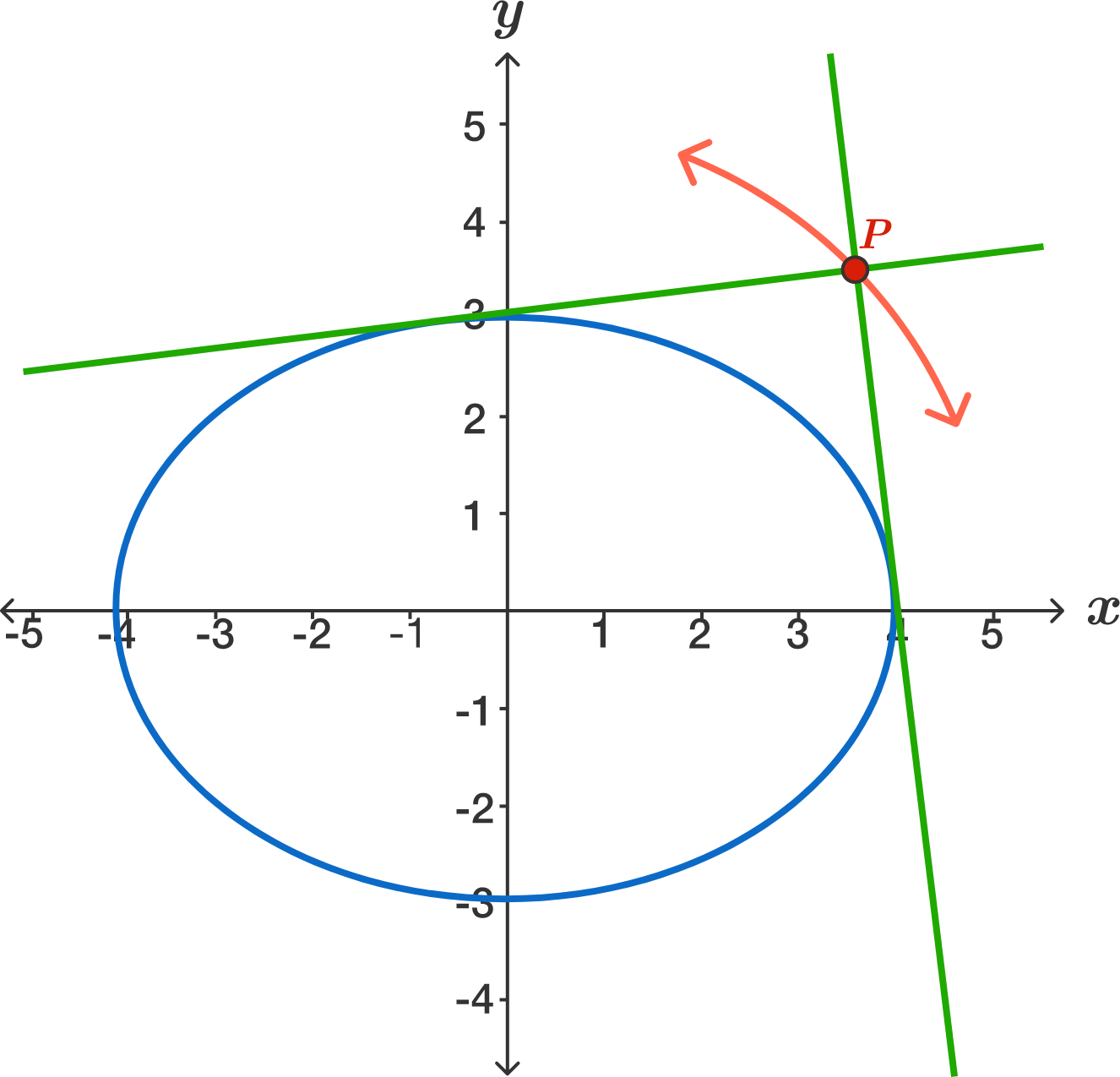# Ellipse and the Right Angle

Geometry Level 4

Below is the graph of the ellipse $\large\frac{x^2}{16}+\frac{y^2}{9}=1$ and two lines that are tangent to the ellipse and meet at right angles at a point $P.$ If we trace out the path of $P,$ its total length can be expressed as $k\pi.$ What is $k?$×

Problem Loading...

Note Loading...

Set Loading...